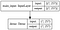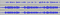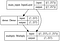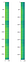# Let’s make something by ourselves

## Basic idea

We want to create a model which predict the gain to apply to noisy frequencies to close clear frequencies.

We use STFT to obtain spectrogram of the input, then we predict and apply the gain to it, and finally we use inverse STFT to reconstruct a waveform.

## Waveform to Spectrogram

`def audioToTensor(filepath:str):    audio_binary = tf.io.read_file(filepath)    audio, audioSR = tf.audio.decode_wav(audio_binary)    audioSR = tf.get_static_value(audioSR)    audio = tf.squeeze(audio, axis=-1)    frame_step = int(audioSR * 0.008)#16000*0.008=128    spectrogram = tf.signal.stft(audio, frame_length=frame_length, frame_step=frame_step)#->31hz, si 512 -> 64hz    spect_image = tf.math.imag(spectrogram)    spect_real = tf.math.real(spectrogram)    spect_sign = tf.sign(spect_real)    spect_real = tf.abs(spect_real)    return spect_real, spect_image, spect_sign, audioSR`

## Spectrogram to Waveform

`def spectToOscillo(spect_real, spect_sign, spect_image, audioSR):    frame_step = int(audioSR * 0.008)    spect_real = pow(10, spect_real/20)#power value    spect_real*=spect_sign    spect_all = tf.complex(spect_real, spect_image)    inverse_stft = tf.signal.inverse_stft(spect_all, frame_length=frame_length, frame_step=frame_step, window_fn=tf.signal.inverse_stft_window_fn(frame_step))    return inverse_stft`

## Create data

In order to create data, we add noise to clear data.

## Load data

Since we need the original input data in the fitting loss function, then we will append it to the clear data.

`testSpect, _, _, _ = audioToTensor('data/noisy/book_00000_chp_0009_reader_06709_0_---1_cCGK4M.wav')print("testSpect.shape:", testSpect.shape)max = 3000x_train = np.zeros((max, voice_max_length, test.shape))y_train = np.zeros((max, v.shape*2))data_count = 0for i, path_clear in enumerate(clear_files):    path_noisy = path_clear.replace("clear", "noisy")    spectNoisy, _, _, _ = audioToTensor(path_noisy)    spectClear, _, _, _ = audioToTensor(path_clear)    for k in range(0, len(spectNoisy)-voice_max_length):        x_train[data_count] = spectNoisy[k:k+voice_max_length]        y_train[data_count] = np.append(spectClear[k+voice_max_length], spectNoisy[k+voice_max_length])        data_count+=1        if data_count>=max:            break    if data_count>=max:        break`

## Prepare some models

First as baseline, we create a Single Dense layer, to predict clear frequencies from noisy one. This is not a good solution, since it can completely change the output and lose meaningful frequencies.Single Dense Layer
`main_input = Input(shape=(int(frame_length/2+1)), name='main_input')x = main_inputx = Dense(int(frame_length/2+1), activation='linear')(x)model = Model(inputs=main_input, outputs=x)`

On below screenshot, we can see that the clear, noisy and predict sound waveform.1) Clear 2) Noisy 3) Predict

Second, we create a Single Dense Gain layer to predict the gain/coefficient to apply to input noisy frequencies to close clear one.Gain predict model
`main_input = Input(shape=(int(frame_length/2+1)), name='main_input')x = main_inputx = Dense(int(frame_length/2+1), activation='sigmoid')(x)x = Multiply()([x, main_input])model = Model(inputs=main_input, outputs=x)`

Third, we create a 2dims Dense Gain model to predict gain to apply to frequencies for a period of time. Input x-axis represents frequencies and y-axis represents time.

`main_input = Input(shape=(image_width, int(frame_length/2+1)), name='main_input')x = main_inputx = Reshape((image_width, int(frame_length/2+1), 1))(x)x = preprocessing.Resizing(image_width//2, int(frame_length/2+1)//2)(x)x = Conv2D(34, 3, activation='relu')(x)x = Conv2D(64, 3, activation='relu')(x)x = MaxPooling2D()(x)x = Dropout(0.1)(x)x = Flatten()(x)x = Dense(int(frame_length/2+1), activation='sigmoid')(x)x = Multiply()([x, main_input])model = Model(inputs=main_input, outputs=x)`

Forth, we use LSTM to predict the gain.

`main_input = Input(shape=(None, int(frame_length/2+1)), name='main_input')x = main_inputx = LSTM(256, activation='tanh', recurrent_activation='sigmoid', return_sequences=False)(x)x = Dense(int(frame_length/2+1), activation='sigmoid')(x)x = Multiply()([x, main_input[:,-1]])model = Model(inputs=main_input, outputs=x)`

We try with LSTM Sequence too.

`main_input = Input(shape=(None, int(frame_length/2+1)), name='main_input')x = main_inputx = LSTM(256, activation='tanh', recurrent_activation='sigmoid', return_sequences=True)(x)x = Dense(int(frame_length/2+1), activation='sigmoid')(x)x = Multiply()([x, main_input])model = Model(inputs=main_input, outputs=x)`

Fifth, we combine all to create LSTM 2dims model.

`main_input = Input(shape=(voice_max_length, parts.shape, parts.shape), name='main_input')x = main_inputx = TimeDistributed(Reshape((image_width, int(frame_length/2+1), 1)))(x)x = TimeDistributed(preprocessing.Resizing(image_width//4, parts.shape//4))(x)x = TimeDistributed(Conv2D(34, 3, activation='relu'))(x)x = TimeDistributed(Conv2D(64, 3, activation='relu'))(x)x = TimeDistributed(MaxPooling2D())(x)x = TimeDistributed(Dropout(0.1))(x)x = TimeDistributed(Flatten())(x)x = LSTM(256, activation='tanh', recurrent_activation='sigmoid', return_sequences=True)(x)x = Dense(int(frame_length/2+1), activation='sigmoid')(x)x = Reshape((voice_max_length, 1, 257))(x)x = Multiply()([x, main_input])model = Model(inputs=main_input, outputs=x)`

## General precision calculation

`print('Evaluate...')total_loss = 0for i, path_clear in enumerate(clear_files):    path_noisy = path_clear.replace("clear", "noisy")    spectNoisy, _, _, _ = audioToTensor(path_noisy)    spectClear, _, _, _ = audioToTensor(path_clear)    result = model.predict(spectNoisy)    loss = np.mean(tf.keras.losses.mean_squared_error(spectClear, result).numpy())    total_loss+=loss    print(path_noisy, "->", loss)print("total_loss:", total_loss/len(clear_files))`

## Example of spectrogramLeft: Input Right: Output

# Possible improvements

As used in RNNoise, we could use Bark scale to reduce input size.

Working in computer science.

## More from Arnaud

Working in computer science.